IdentifiantMot de passe
Mot de passe oublié ?

Inscrivez-vous gratuitement
pour pouvoir participer, suivre les réponses en temps réel, voter pour les messages, poser vos propres questions et recevoir la newsletter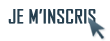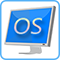Discussion :

# comment reprogrammer la partie animation du noyau ?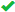Sujet :

## Cpcdos

1.comment reprogrammer la partie animation du noyau ?
bonsoir j'aimerais savoir si pour reprogrammer la partie animation du noyau il faut bien modifier le fichier krnl_dem quand je modifie pour changer mon boot
avoir un boot animé a la place de l'image "votre ecrant de demarrage" il me defile mes image avant il maffiche quand meme cette image et se coupe et avec d'autre essai il me lance le kernel apres il me met l'image qui me ser de boot pui limage "votre ecrant de demarrage" et le bureau alors que moi j'aimerais changer l'image "votre ecrant de demarrage" pour mettre un boot animer soit gif soit plusieurs imageboxRépondre avec citation   0  0

2.Animation au bootscreen
Bonsoir,

je te conseille de contacter BlackoOSx si je ne me trompe pas..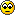Il nous confirmera!
il a réussit cet exploit que même moi je pensais pas qu'il était possible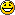Le voici en vidéo :Répondre avec citation   0  0

3.je m'étais déja inspiré de lui mais le mien je ne sais pas pourquoi il reste figer 17 seconde avant de mafficher mon bureauRépondre avec citation   0  0

4.Salut,
Est-ce que tu peux me faire voire le code de tous les fichiers que tu as modifié pour faire cet écran de démarrage animé.
Peut-être que je pourrais t'aider car c'est moi qui a fait cette vidéo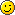bien que la version 3.9 ainsi que cette vidéo sont très obsolètes et il y a sûrement des erreurs que j'ai fait dans cette vidéo!Répondre avec citation   0  0

5.voici le code de index.cpc qui se trouve dans INIT:
 Code : Sélectionner tout - Visualiser dans une fenêtre à part
```12345678910111213141516171819202122232425262728293031rem/ Microsf01 Cpcdos OS2
rem/ Indexeur de l'initialisation.
rem/ Les commandes comme pause ne servent qu'a mettre en pause le systeme et voir ce qui defile a l'ecran, pour vous..
fix/ log = 0
fix/ DEBUG = 1
fix/ STOP_ERREUR = 0
fix/ BOOTOS = OS\INDEX.CPC /l:Autoexec

Rem/ Si INIT_AFFICHAGE = 1 alors il initialisera la liste des affichages 16, 24 et 32bits dans ces tableaux
rem/ %RESOLUTIONS_16(x)%       %RESOLUTIONS_16% = Nombre resolutions 16bits
rem/ %RESOLUTIONS_24(x)%       %RESOLUTIONS_24% = Nombre resolutions 24bits
rem/ %RESOLUTIONS_32(x)%       %RESOLUTIONS_32% = Nombre resolutions 32bits
Rem/ Si INIT_AFFICHAGE = 0 alors il ne le fera pas, et chargement et plus rapide !

fix/ INIT_AFFICHAGE = 0

IUG/
EXE/ & INIT\KRNL_DEM.CPC

:_KRNL_CFG:
exe/ & INIT\KRNL_CFG.CPC /l:_CFG

:_KRNL_INI:
fix/ STOP_ERREUR = 1
exe/ & INIT\KRNL_INI.CPC /l:_INI_1

:_OS:
Txt/ /debug Kernel : Execution du serveur d'hebergement des services locaux...
EXE/ & KRNL\SRV_SVC.CPC

demarrer/```
et celui de krnl_dem:

 Code : Sélectionner tout - Visualiser dans une fenêtre à part
```123456789101112131415161718192021222324252627282930313233343536373839404142434445464748495051525354555657585960616263646566676869707172737475767778798081828384858687888990919293949596979899100101102103104105106107108109110111112113114115116117118119120121122123124125126127128129130131132133134135136137138139140141142143144145146147148149150151152153154155156157158159160161162163164165166167168169170171172173174175176177178179180181182183184185186187188189190191192193194195196197198199200201202203204205206207208209210211212213214215216217218219220221222223224225226227228229230231232233234235236237238239240241242243244245246247248249250251252253254255256257258259260261262263264265266267268269270271272273274275276277278279280281282283284285286287288289290291292293294295296297298299300301302303304305306307308309310311312313314315316317318319320321322323324325326327328329330331332333334335336337338339340341342343344345346347348349350351352353354355356357358359360361362363364365366367368369370371372373374375376377378379380381382383384385386387388389390391392393394395396397398399400401402403404405406407408409410411412413414415416417418419420421422423424425426427428429430431432433434435436437438439440441442443444445446447448449450451452453454455456457458459460461462463464465466467468469470471472473474475476477478479480481482483484485486487488489490491492493494495496497498499500501502503504505506507508509510511512513514515516517518519520521522523524525526527528529530531532533534535536537538539540541542543544545546547548549550551552553554555556557558559560561562563564565566567568569570571572573574575576577578579580581582583584585586587588589590591592593594595596597598599600601602603604605606607608609610611612613614615616617618619620621622623624625626627628629630631632633634635636637638639640641642643644645646647648649650651652653654655656657658659660661662663664665666667668669670671672673674675676677678679680681682683684685686687688689690691692693694695696697698699700701702703704705706707708709710711712713714715716717718719720721722723724725726727728729730731732733734735736737738739740741742743744745746747748749750751752fix/ anim_COULEUR_F_R = 75
fix/ anim_COULEUR_F_V = 75
fix/ anim_COULEUR_F_B = 75
FIX/ anim_COULEUR_P_R = 200
FIX/ anim_COULEUR_P_V = 200
FIX/ anim_COULEUR_P_B = 200
FIX/ anim_COULEUR_OMBRE = 50
FIX/ anim_BAS = AUTO N16
fix/ anim_TYPE_BARRE = 0
fix/ animATION = 0

LC/
IUG/
INI/ Fenetre(
INI;NOM         = "ANIM"
INI;TYPE        = "3;M0A0R0F0T0C0O0B0BC0"
INI;PX          = "0"
INI;PY          = "0"
INI;TX          = "800"
INI;TY          = "600"
CREER/
INI/ Fenetre)

DEMARRER/

INI/ Imagebox(
INI;NOM         = "IMG_ANIM"
INI;FENETRE     = "ANIM"
INI;TYPE        = "0"
INI;IMAGE       = "OS\MEDIA\FOND\DEM.BMP"
INI;PX          = "0"
INI;PY          = "0"
INI;TX          = "800"
INI;TY          = "600"
INI;COULEUR 	= "000,000,000"
INI;COULEURF 	= "000,000,000"
CREER/
INI/ Imagebox)

FIX/ PX = 400
FIX/ PY = 300

:ANIM:

INI/ Imagebox(
INI;NOM         = "IMG_POINTS"
INI;FENETRE     = "ANIM"
INI;TYPE        = "0"
INI;IMAGE       = "OS\MEDIA\FOND\DEM\DEM01.BMP"
INI;PX          = "%PX%"
INI;PY          = "%PY%"
INI;TX          = "150"
INI;TY          = "150"
INI;COULEUR 	= "000,000,000"
INI;COULEURF 	= "000,000,000"
CREER/
INI/ Imagebox)

rem/ #1

INI/ Compteur(
INI;NOM         = "ANIM_BAR"
INI;FENETRE     = "0.100"
INI;ACTIVE      = "1"
CREER/
INI/ Compteur)

INI/ Imagebox(
INI;NOM         = "IMG_POINTS"
INI;FENETRE     = "ANIM"
INI;TYPE        = "0"
INI;IMAGE       = "OS\MEDIA\FOND\DEM\DEM02.BMP"
INI;PX          = "%PX%"
INI;PY          = "%PY%"
INI;TX          = "150"
INI;TY          = "150"
INI;COULEUR 	= "000,000,000"
INI;COULEURF 	= "000,000,000"
CREER/
INI/ Imagebox)

INI/ Compteur(
INI;NOM         = "ANIM_BAR"
INI;FENETRE     = "0.100"
INI;ACTIVE      = "1"
CREER/
INI/ Compteur)

INI/ Imagebox(
INI;NOM         = "IMG_POINTS"
INI;FENETRE     = "ANIM"
INI;TYPE        = "0"
INI;IMAGE       = "OS\MEDIA\FOND\DEM\DEM03.BMP"
INI;PX          = "%PX%"
INI;PY          = "%PY%"
INI;TX          = "150"
INI;TY          = "150"
INI;COULEUR 	= "000,000,000"
INI;COULEURF 	= "000,000,000"
CREER/
INI/ Imagebox)

INI/ Compteur(
INI;NOM         = "ANIM_BAR"
INI;FENETRE     = "0.100"
INI;ACTIVE      = "1"
CREER/
INI/ Compteur)

INI/ Imagebox(
INI;NOM         = "IMG_POINTS"
INI;FENETRE     = "ANIM"
INI;TYPE        = "0"
INI;IMAGE       = "OS\MEDIA\FOND\DEM\DEM04.BMP"
INI;PX          = "%PX%"
INI;PY          = "%PY%"
INI;TX          = "150"
INI;TY          = "150"
INI;COULEUR 	= "000,000,000"
INI;COULEURF 	= "000,000,000"
CREER/
INI/ Imagebox)

INI/ Compteur(
INI;NOM         = "ANIM_BAR"
INI;FENETRE     = "0.100"
INI;ACTIVE      = "1"
CREER/
INI/ Compteur)

INI/ Imagebox(
INI;NOM         = "IMG_POINTS"
INI;FENETRE     = "ANIM"
INI;TYPE        = "0"
INI;IMAGE       = "OS\MEDIA\FOND\DEM\DEM05.BMP"
INI;PX          = "%PX%"
INI;PY          = "%PY%"
INI;TX          = "150"
INI;TY          = "150"
INI;COULEUR 	= "000,000,000"
INI;COULEURF 	= "000,000,000"
CREER/
INI/ Imagebox)

INI/ Compteur(
INI;NOM         = "ANIM_BAR"
INI;FENETRE     = "0.100"
INI;ACTIVE      = "1"
CREER/
INI/ Compteur)

INI/ Imagebox(
INI;NOM         = "IMG_POINTS"
INI;FENETRE     = "ANIM"
INI;TYPE        = "0"
INI;IMAGE       = "OS\MEDIA\FOND\DEM\DEM06.BMP"
INI;PX          = "%PX%"
INI;PY          = "%PY%"
INI;TX          = "150"
INI;TY          = "150"
INI;COULEUR 	= "000,000,000"
INI;COULEURF 	= "000,000,000"
CREER/
INI/ Imagebox)

INI/ Compteur(
INI;NOM         = "ANIM_BAR"
INI;FENETRE     = "0.100"
INI;ACTIVE      = "1"
CREER/
INI/ Compteur)

INI/ Imagebox(
INI;NOM         = "IMG_POINTS"
INI;FENETRE     = "ANIM"
INI;TYPE        = "0"
INI;IMAGE       = "OS\MEDIA\FOND\DEM\DEM07.BMP"
INI;PX          = "%PX%"
INI;PY          = "%PY%"
INI;TX          = "150"
INI;TY          = "150"
INI;COULEUR 	= "000,000,000"
INI;COULEURF 	= "000,000,000"
CREER/
INI/ Imagebox)

INI/ Compteur(
INI;NOM         = "ANIM_BAR"
INI;FENETRE     = "0.100"
INI;ACTIVE      = "1"
CREER/
INI/ Compteur)

INI/ Imagebox(
INI;NOM         = "IMG_POINTS"
INI;FENETRE     = "ANIM"
INI;TYPE        = "0"
INI;IMAGE       = "OS\MEDIA\FOND\DEM\DEM08.BMP"
INI;PX          = "%PX%"
INI;PY          = "%PY%"
INI;TX          = "150"
INI;TY          = "150"
INI;COULEUR 	= "000,000,000"
INI;COULEURF 	= "000,000,000"
CREER/
INI/ Imagebox)

INI/ Compteur(
INI;NOM         = "ANIM_BAR"
INI;FENETRE     = "0.100"
INI;ACTIVE      = "1"
CREER/
INI/ Compteur)

INI/ Imagebox(
INI;NOM         = "IMG_POINTS"
INI;FENETRE     = "ANIM"
INI;TYPE        = "0"
INI;IMAGE       = "OS\MEDIA\FOND\DEM\DEM09.BMP"
INI;PX          = "%PX%"
INI;PY          = "%PY%"
INI;TX          = "150"
INI;TY          = "150"
INI;COULEUR 	= "000,000,000"
INI;COULEURF 	= "000,000,000"
CREER/
INI/ Imagebox)

INI/ Compteur(
INI;NOM         = "ANIM_BAR"
INI;FENETRE     = "0.100"
INI;ACTIVE      = "1"
CREER/
INI/ Compteur)

INI/ Imagebox(
INI;NOM         = "IMG_POINTS"
INI;FENETRE     = "ANIM"
INI;TYPE        = "0"
INI;IMAGE       = "OS\MEDIA\FOND\DEM\DEM10.BMP"
INI;PX          = "%PX%"
INI;PY          = "%PY%"
INI;TX          = "150"
INI;TY          = "150"
INI;COULEUR 	= "000,000,000"
INI;COULEURF 	= "000,000,000"
CREER/
INI/ Imagebox)

INI/ Compteur(
INI;NOM         = "ANIM_BAR"
INI;FENETRE     = "0.100"
INI;ACTIVE      = "1"
CREER/
INI/ Compteur)

INI/ Imagebox(
INI;NOM         = "IMG_POINTS"
INI;FENETRE     = "ANIM"
INI;TYPE        = "0"
INI;IMAGE       = "OS\MEDIA\FOND\DEM\DEM11.BMP"
INI;PX          = "%PX%"
INI;PY          = "%PY%"
INI;TX          = "150"
INI;TY          = "150"
INI;COULEUR 	= "000,000,000"
INI;COULEURF 	= "000,000,000"
CREER/
INI/ Imagebox)

INI/ Compteur(
INI;NOM         = "ANIM_BAR"
INI;FENETRE     = "0.100"
INI;ACTIVE      = "1"
CREER/
INI/ Compteur)

INI/ Imagebox(
INI;NOM         = "IMG_POINTS"
INI;FENETRE     = "ANIM"
INI;TYPE        = "0"
INI;IMAGE       = "OS\MEDIA\FOND\DEM\DEM12.BMP"
INI;PX          = "%PX%"
INI;PY          = "%PY%"
INI;TX          = "150"
INI;TY          = "150"
INI;COULEUR 	= "000,000,000"
INI;COULEURF 	= "000,000,000"
CREER/
INI/ Imagebox)

INI/ Compteur(
INI;NOM         = "ANIM_BAR"
INI;FENETRE     = "0.100"
INI;ACTIVE      = "1"
CREER/
INI/ Compteur)

INI/ Imagebox(
INI;NOM         = "IMG_POINTS"
INI;FENETRE     = "ANIM"
INI;TYPE        = "0"
INI;IMAGE       = "OS\MEDIA\FOND\DEM\DEM13.BMP"
INI;PX          = "%PX%"
INI;PY          = "%PY%"
INI;TX          = "150"
INI;TY          = "150"
INI;COULEUR 	= "000,000,000"
INI;COULEURF 	= "000,000,000"
CREER/
INI/ Imagebox)

INI/ Compteur(
INI;NOM         = "ANIM_BAR"
INI;FENETRE     = "0.100"
INI;ACTIVE      = "1"
CREER/
INI/ Compteur)

INI/ Imagebox(
INI;NOM         = "IMG_POINTS"
INI;FENETRE     = "ANIM"
INI;TYPE        = "0"
INI;IMAGE       = "OS\MEDIA\FOND\DEM\DEM14.BMP"
INI;PX          = "%PX%"
INI;PY          = "%PY%"
INI;TX          = "150"
INI;TY          = "150"
INI;COULEUR 	= "000,000,000"
INI;COULEURF 	= "000,000,000"
CREER/
INI/ Imagebox)

INI/ Compteur(
INI;NOM         = "ANIM_BAR"
INI;FENETRE     = "0.100"
INI;ACTIVE      = "1"
CREER/
INI/ Compteur)

INI/ Imagebox(
INI;NOM         = "IMG_POINTS"
INI;FENETRE     = "ANIM"
INI;TYPE        = "0"
INI;IMAGE       = "OS\MEDIA\FOND\DEM\DEM15.BMP"
INI;PX          = "%PX%"
INI;PY          = "%PY%"
INI;TX          = "150"
INI;TY          = "150"
INI;COULEUR 	= "000,000,000"
INI;COULEURF 	= "000,000,000"
CREER/
INI/ Imagebox)

INI/ Compteur(
INI;NOM         = "ANIM_BAR"
INI;FENETRE     = "0.100"
INI;ACTIVE      = "1"
CREER/
INI/ Compteur)

INI/ Imagebox(
INI;NOM         = "IMG_POINTS"
INI;FENETRE     = "ANIM"
INI;TYPE        = "0"
INI;IMAGE       = "OS\MEDIA\FOND\DEM\DEM16.BMP"
INI;PX          = "%PX%"
INI;PY          = "%PY%"
INI;TX          = "150"
INI;TY          = "150"
INI;COULEUR 	= "000,000,000"
INI;COULEURF 	= "000,000,000"
CREER/
INI/ Imagebox)

INI/ Compteur(
INI;NOM         = "ANIM_BAR"
INI;FENETRE     = "0.100"
INI;ACTIVE      = "1"
CREER/
INI/ Compteur)

INI/ Imagebox(
INI;NOM         = "IMG_POINTS"
INI;FENETRE     = "ANIM"
INI;TYPE        = "0"
INI;IMAGE       = "OS\MEDIA\FOND\DEM\DEM17.BMP"
INI;PX          = "%PX%"
INI;PY          = "%PY%"
INI;TX          = "150"
INI;TY          = "150"
INI;COULEUR 	= "000,000,000"
INI;COULEURF 	= "000,000,000"
CREER/
INI/ Imagebox)
INI/ Compteur(
INI;NOM         = "ANIM_BAR"
INI;FENETRE     = "0.100"
INI;ACTIVE      = "1"
CREER/
INI/ Compteur)

INI/ Imagebox(
INI;NOM         = "IMG_POINTS"
INI;FENETRE     = "ANIM"
INI;TYPE        = "0"
INI;IMAGE       = "OS\MEDIA\FOND\DEM\DEM01.BMP"
INI;PX          = "%PX%"
INI;PY          = "%PY%"
INI;TX          = "150"
INI;TY          = "150"
INI;COULEUR 	= "000,000,000"
INI;COULEURF 	= "000,000,000"
CREER/
INI/ Imagebox)

INI/ Compteur(
INI;NOM         = "ANIM_BAR"
INI;FENETRE     = "0.100"
INI;ACTIVE      = "1"
CREER/
INI/ Compteur)

INI/ Imagebox(
INI;NOM         = "IMG_POINTS"
INI;FENETRE     = "ANIM"
INI;TYPE        = "0"
INI;IMAGE       = "OS\MEDIA\FOND\DEM\DEM02.BMP"
INI;PX          = "%PX%"
INI;PY          = "%PY%"
INI;TX          = "150"
INI;TY          = "150"
INI;COULEUR 	= "000,000,000"
INI;COULEURF 	= "000,000,000"
CREER/
INI/ Imagebox)

INI/ Compteur(
INI;NOM         = "ANIM_BAR"
INI;FENETRE     = "0.100"
INI;ACTIVE      = "1"
CREER/
INI/ Compteur)

INI/ Imagebox(
INI;NOM         = "IMG_POINTS"
INI;FENETRE     = "ANIM"
INI;TYPE        = "0"
INI;IMAGE       = "OS\MEDIA\FOND\DEM\DEM03.BMP"
INI;PX          = "%PX%"
INI;PY          = "%PY%"
INI;TX          = "150"
INI;TY          = "150"
INI;COULEUR 	= "000,000,000"
INI;COULEURF 	= "000,000,000"
CREER/
INI/ Imagebox)

INI/ Compteur(
INI;NOM         = "ANIM_BAR"
INI;FENETRE     = "0.100"
INI;ACTIVE      = "1"
CREER/
INI/ Compteur)

INI/ Imagebox(
INI;NOM         = "IMG_POINTS"
INI;FENETRE     = "ANIM"
INI;TYPE        = "0"
INI;IMAGE       = "OS\MEDIA\FOND\DEM\DEM04.BMP"
INI;PX          = "%PX%"
INI;PY          = "%PY%"
INI;TX          = "150"
INI;TY          = "150"
INI;COULEUR 	= "000,000,000"
INI;COULEURF 	= "000,000,000"
CREER/
INI/ Imagebox)

INI/ Compteur(
INI;NOM         = "ANIM_BAR"
INI;FENETRE     = "0.100"
INI;ACTIVE      = "1"
CREER/
INI/ Compteur)

INI/ Imagebox(
INI;NOM         = "IMG_POINTS"
INI;FENETRE     = "ANIM"
INI;TYPE        = "0"
INI;IMAGE       = "OS\MEDIA\FOND\DEM\DEM05.BMP"
INI;PX          = "%PX%"
INI;PY          = "%PY%"
INI;TX          = "150"
INI;TY          = "150"
INI;COULEUR 	= "000,000,000"
INI;COULEURF 	= "000,000,000"
CREER/
INI/ Imagebox)

INI/ Compteur(
INI;NOM         = "ANIM_BAR"
INI;FENETRE     = "0.100"
INI;ACTIVE      = "1"
CREER/
INI/ Compteur)

INI/ Imagebox(
INI;NOM         = "IMG_POINTS"
INI;FENETRE     = "ANIM"
INI;TYPE        = "0"
INI;IMAGE       = "OS\MEDIA\FOND\DEM\DEM06.BMP"
INI;PX          = "%PX%"
INI;PY          = "%PY%"
INI;TX          = "150"
INI;TY          = "150"
INI;COULEUR 	= "000,000,000"
INI;COULEURF 	= "000,000,000"
CREER/
INI/ Imagebox)

INI/ Compteur(
INI;NOM         = "ANIM_BAR"
INI;FENETRE     = "0.100"
INI;ACTIVE      = "1"
CREER/
INI/ Compteur)

INI/ Imagebox(
INI;NOM         = "IMG_POINTS"
INI;FENETRE     = "ANIM"
INI;TYPE        = "0"
INI;IMAGE       = "OS\MEDIA\FOND\DEM\DEM07.BMP"
INI;PX          = "%PX%"
INI;PY          = "%PY%"
INI;TX          = "150"
INI;TY          = "150"
INI;COULEUR 	= "000,000,000"
INI;COULEURF 	= "000,000,000"
CREER/
INI/ Imagebox)

INI/ Compteur(
INI;NOM         = "ANIM_BAR"
INI;FENETRE     = "0.100"
INI;ACTIVE      = "1"
CREER/
INI/ Compteur)

INI/ Imagebox(
INI;NOM         = "IMG_POINTS"
INI;FENETRE     = "ANIM"
INI;TYPE        = "0"
INI;IMAGE       = "OS\MEDIA\FOND\DEM\DEM08.BMP"
INI;PX          = "%PX%"
INI;PY          = "%PY%"
INI;TX          = "150"
INI;TY          = "150"
INI;COULEUR 	= "000,000,000"
INI;COULEURF 	= "000,000,000"
CREER/
INI/ Imagebox)

INI/ Compteur(
INI;NOM         = "ANIM_BAR"
INI;FENETRE     = "0.100"
INI;ACTIVE      = "1"
CREER/
INI/ Compteur)

INI/ Imagebox(
INI;NOM         = "IMG_POINTS"
INI;FENETRE     = "ANIM"
INI;TYPE        = "0"
INI;IMAGE       = "OS\MEDIA\FOND\DEM\DEM09.BMP"
INI;PX          = "%PX%"
INI;PY          = "%PY%"
INI;TX          = "150"
INI;TY          = "150"
INI;COULEUR 	= "000,000,000"
INI;COULEURF 	= "000,000,000"
CREER/
INI/ Imagebox)

INI/ Compteur(
INI;NOM         = "ANIM_BAR"
INI;FENETRE     = "0.100"
INI;ACTIVE      = "1"
CREER/
INI/ Compteur)

INI/ Imagebox(
INI;NOM         = "IMG_POINTS"
INI;FENETRE     = "ANIM"
INI;TYPE        = "0"
INI;IMAGE       = "OS\MEDIA\FOND\DEM\DEM10.BMP"
INI;PX          = "%PX%"
INI;PY          = "%PY%"
INI;TX          = "150"
INI;TY          = "150"
INI;COULEUR 	= "000,000,000"
INI;COULEURF 	= "000,000,000"
CREER/
INI/ Imagebox)

INI/ Compteur(
INI;NOM         = "ANIM_BAR"
INI;FENETRE     = "0.100"
INI;ACTIVE      = "1"
CREER/
INI/ Compteur)

INI/ Imagebox(
INI;NOM         = "IMG_POINTS"
INI;FENETRE     = "ANIM"
INI;TYPE        = "0"
INI;IMAGE       = "OS\MEDIA\FOND\DEM\DEM11.BMP"
INI;PX          = "%PX%"
INI;PY          = "%PY%"
INI;TX          = "150"
INI;TY          = "150"
INI;COULEUR 	= "000,000,000"
INI;COULEURF 	= "000,000,000"
CREER/
INI/ Imagebox)

INI/ Compteur(
INI;NOM         = "ANIM_BAR"
INI;FENETRE     = "0.100"
INI;ACTIVE      = "1"
CREER/
INI/ Compteur)

INI/ Imagebox(
INI;NOM         = "IMG_POINTS"
INI;FENETRE     = "ANIM"
INI;TYPE        = "0"
INI;IMAGE       = "OS\MEDIA\FOND\DEM\DEM12.BMP"
INI;PX          = "%PX%"
INI;PY          = "%PY%"
INI;TX          = "150"
INI;TY          = "150"
INI;COULEUR 	= "000,000,000"
INI;COULEURF 	= "000,000,000"
CREER/
INI/ Imagebox)

INI/ Compteur(
INI;NOM         = "ANIM_BAR"
INI;FENETRE     = "0.100"
INI;ACTIVE      = "1"
CREER/
INI/ Compteur)

INI/ Imagebox(
INI;NOM         = "IMG_POINTS"
INI;FENETRE     = "ANIM"
INI;TYPE        = "0"
INI;IMAGE       = "OS\MEDIA\FOND\DEM\DEM13.BMP"
INI;PX          = "%PX%"
INI;PY          = "%PY%"
INI;TX          = "150"
INI;TY          = "150"
INI;COULEUR 	= "000,000,000"
INI;COULEURF 	= "000,000,000"
CREER/
INI/ Imagebox)

INI/ Compteur(
INI;NOM         = "ANIM_BAR"
INI;FENETRE     = "0.100"
INI;ACTIVE      = "1"
CREER/
INI/ Compteur)

INI/ Imagebox(
INI;NOM         = "IMG_POINTS"
INI;FENETRE     = "ANIM"
INI;TYPE        = "0"
INI;IMAGE       = "OS\MEDIA\FOND\DEM\DEM14.BMP"
INI;PX          = "%PX%"
INI;PY          = "%PY%"
INI;TX          = "150"
INI;TY          = "150"
INI;COULEUR 	= "000,000,000"
INI;COULEURF 	= "000,000,000"
CREER/
INI/ Imagebox)

INI/ Compteur(
INI;NOM         = "ANIM_BAR"
INI;FENETRE     = "0.100"
INI;ACTIVE      = "1"
CREER/
INI/ Compteur)

INI/ Imagebox(
INI;NOM         = "IMG_POINTS"
INI;FENETRE     = "ANIM"
INI;TYPE        = "0"
INI;IMAGE       = "OS\MEDIA\FOND\DEM\DEM15.BMP"
INI;PX          = "%PX%"
INI;PY          = "%PY%"
INI;TX          = "150"
INI;TY          = "150"
INI;COULEUR 	= "000,000,000"
INI;COULEURF 	= "000,000,000"
CREER/
INI/ Imagebox)

INI/ Compteur(
INI;NOM         = "ANIM_BAR"
INI;FENETRE     = "0.100"
INI;ACTIVE      = "1"
CREER/
INI/ Compteur)

INI/ Imagebox(
INI;NOM         = "IMG_POINTS"
INI;FENETRE     = "ANIM"
INI;TYPE        = "0"
INI;IMAGE       = "OS\MEDIA\FOND\DEM\DEM16.BMP"
INI;PX          = "%PX%"
INI;PY          = "%PY%"
INI;TX          = "150"
INI;TY          = "150"
INI;COULEUR 	= "000,000,000"
INI;COULEURF 	= "000,000,000"
CREER/
INI/ Imagebox)

INI/ Compteur(
INI;NOM         = "ANIM_BAR"
INI;FENETRE     = "0.100"
INI;ACTIVE      = "1"
CREER/
INI/ Compteur)

INI/ Imagebox(
INI;NOM         = "IMG_POINTS"
INI;FENETRE     = "ANIM"
INI;TYPE        = "0"
INI;IMAGE       = "OS\MEDIA\FOND\DEM\DEM17.BMP"
INI;PX          = "%PX%"
INI;PY          = "%PY%"
INI;TX          = "150"
INI;TY          = "150"
INI;COULEUR 	= "000,000,000"
INI;COULEURF 	= "000,000,000"
CREER/
INI/ Imagebox)```Répondre avec citation   0  0

6.Salut,
Regarde avec ce code je l'ai juste un peu modifié mais sinon c'est le tiens:
Dans INDEX.CPC:
 Code : Sélectionner tout - Visualiser dans une fenêtre à part
```123456789101112131415161718192021222324252627FIX/ log = 0FIX/ DEBUG = 1
FIX/ STOP_ERREUR = 0
FIX/ BOOTOS = OS\INDEX.CPC /l:Autoexec

FIX/ INIT_AFFICHAGE = 0

IUG/
EXE/ & INIT\KRNL_DEM.CPC

:_KRNL_CFG:
EXE/ & INIT\KRNL_CFG.CPC /l:_CFG

:_KRNL_INI:
FIX/ STOP_ERREUR = 1
EXE/ & INIT\KRNL_INI.CPC /l:_INI_1

:_OS:
Txt/ /debug Kernel : Execution du serveur d'hebergement des services locaux...
EXE/ & KRNL\SRV_SVC.CPC

DEMARRER/```
Et dans KRNL_DEM.CPC:
 Code : Sélectionner tout - Visualiser dans une fenêtre à part
```123456789101112131415161718192021222324252627282930313233343536373839404142434445464748495051525354555657585960616263646566676869707172737475767778798081828384858687888990919293949596979899100101102103104105106107108109110111112113114115116117118119120121122123124125126127128129130131132133134135136137138139140141142143144145146147148149150151152153154155156157158159160161162163164165166167168169170171172173174175176177178179180181182183184185186187188189190191192193194195196197198199200201202203204205206207208209210211212213214215216217218219220221222223224225226227228229230231232233234235236237238239240241242243244245246247248249250251252253254255256257258259260261262263264265266267268269270271272273274275276277278279280281282283284285286287288289290291292293294295296297298299300301302303304305306307308309310311312313314315316317318319320321322323324325326327328329330331332333334335336337338339340341342343344345346347348349350351352353354355356357358359360361362363364365366367368369370371372373374375376377378379380381382383384385386387388389390391392393394395396397398399400401402403404405406407408409410411412413414415416417418419420421422423424425426427428429430431432433434435436437438439440441442443444445446447448449450451452453454455456457458459460461462463464465466467468469470471472473474475476477478479480481482483484485486487488489490491492493494495496497498499500501502503504505506507508509510511512513514515516517518519520521522523524525526527528529530531532533534535536537538539540541542543544545546547548549550551552553554555556557558559560561562563564565566567568569570571572573574575576577578579580581582583584585586587588589590591592593594595596597598599600601602603604605606607608609610611612613614615616617618619620621622623624625626627628629630631632633634635636637638639640641642643644645646647648649650651652653654655656657658659660661662663664665666667668669670671672673674675676677678679680681682683684685686687688689690691692693694695696697698699700701702703704705706707708709710711712713714715716717718719720721722723724725726727728729730731732733734735736737738739740741742743744745746747748749750751752753754755756757758759760761762763764765766767768769770771772773774775776777778779780781782783784785786787788789790791792793794795796797798799800801802803804805806807808809810811812813814815816817818819820821822823FIX/ anim_COULEUR_F_R = 75FIX/ anim_COULEUR_F_V = 75
FIX/ anim_COULEUR_F_B = 75
FIX/ anim_COULEUR_P_R = 200
FIX/ anim_COULEUR_P_V = 200
FIX/ anim_COULEUR_P_B = 200
FIX/ anim_COULEUR_OMBRE = 50
FIX/ anim_BAS = AUTO N16
FIX/ anim_TYPE_BARRE = 0
FIX/ animATION = 0

LC/
IUG/

INI/ Fenetre(
INI;NOM         = "ANIM"
INI;TYPE        = "3;M0A0R0F0T0C0O0B0BC0"
INI;PX          = "0"
INI;PY          = "0"
INI;TX          = "800"
INI;TY          = "600"
CREER/
INI/ Fenetre)

INI/ Imagebox(
INI;NOM         = "IMG_ANIM"
INI;FENETRE     = "ANIM"
INI;TYPE        = "0"
INI;IMAGE       = "OS\MEDIA\FOND\DEM.BMP"
INI;PX          = "0"
INI;PY          = "0"
INI;TX          = "800"
INI;TY          = "600"
INI;COULEUR     = "000,000,000"
INI;COULEURF     = "000,000,000"
CREER/
INI/ Imagebox)

FIX/ PX = 400
FIX/ PY = 300

:ANIM:

INI/ Imagebox(
INI;NOM         = "IMG_POINTS"
INI;FENETRE     = "ANIM"
INI;TYPE        = "0"
INI;IMAGE       = "OS\MEDIA\FOND\DEM\DEM01.BMP"
INI;PX          = "%PX%"
INI;PY          = "%PY%"
INI;TX          = "150"
INI;TY          = "150"
INI;COULEUR     = "000,000,000"
INI;COULEURF     = "000,000,000"
CREER/
INI/ Imagebox)

rem/ #1

INI/ Compteur(
INI;NOM         = "ANIM_BAR"
INI;FENETRE     = "0.100"
INI;ACTIVE      = "1"
CREER/
INI/ Compteur)

INI/ Imagebox(
INI;NOM         = "IMG_POINTS"
INI;FENETRE     = "ANIM"
INI;TYPE        = "0"
INI;IMAGE       = "OS\MEDIA\FOND\DEM\DEM02.BMP"
INI;PX          = "%PX%"
INI;PY          = "%PY%"
INI;TX          = "150"
INI;TY          = "150"
INI;COULEUR     = "000,000,000"
INI;COULEURF     = "000,000,000"
CREER/
INI/ Imagebox)

INI/ Compteur(
INI;NOM         = "ANIM_BAR"
INI;FENETRE     = "0.100"
INI;ACTIVE      = "1"
CREER/
INI/ Compteur)

INI/ Imagebox(
INI;NOM         = "IMG_POINTS"
INI;FENETRE     = "ANIM"
INI;TYPE        = "0"
INI;IMAGE       = "OS\MEDIA\FOND\DEM\DEM03.BMP"
INI;PX          = "%PX%"
INI;PY          = "%PY%"
INI;TX          = "150"
INI;TY          = "150"
INI;COULEUR     = "000,000,000"
INI;COULEURF     = "000,000,000"
CREER/
INI/ Imagebox)

INI/ Compteur(
INI;NOM         = "ANIM_BAR"
INI;FENETRE     = "0.100"
INI;ACTIVE      = "1"
CREER/
INI/ Compteur)

INI/ Imagebox(
INI;NOM         = "IMG_POINTS"
INI;FENETRE     = "ANIM"
INI;TYPE        = "0"
INI;IMAGE       = "OS\MEDIA\FOND\DEM\DEM04.BMP"
INI;PX          = "%PX%"
INI;PY          = "%PY%"
INI;TX          = "150"
INI;TY          = "150"
INI;COULEUR     = "000,000,000"
INI;COULEURF     = "000,000,000"
CREER/
INI/ Imagebox)

INI/ Compteur(
INI;NOM         = "ANIM_BAR"
INI;FENETRE     = "0.100"
INI;ACTIVE      = "1"
CREER/
INI/ Compteur)

INI/ Imagebox(
INI;NOM         = "IMG_POINTS"
INI;FENETRE     = "ANIM"
INI;TYPE        = "0"
INI;IMAGE       = "OS\MEDIA\FOND\DEM\DEM05.BMP"
INI;PX          = "%PX%"
INI;PY          = "%PY%"
INI;TX          = "150"
INI;TY          = "150"
INI;COULEUR     = "000,000,000"
INI;COULEURF     = "000,000,000"
CREER/
INI/ Imagebox)

INI/ Compteur(
INI;NOM         = "ANIM_BAR"
INI;FENETRE     = "0.100"
INI;ACTIVE      = "1"
CREER/
INI/ Compteur)

INI/ Imagebox(
INI;NOM         = "IMG_POINTS"
INI;FENETRE     = "ANIM"
INI;TYPE        = "0"
INI;IMAGE       = "OS\MEDIA\FOND\DEM\DEM06.BMP"
INI;PX          = "%PX%"
INI;PY          = "%PY%"
INI;TX          = "150"
INI;TY          = "150"
INI;COULEUR     = "000,000,000"
INI;COULEURF     = "000,000,000"
CREER/
INI/ Imagebox)

INI/ Compteur(
INI;NOM         = "ANIM_BAR"
INI;FENETRE     = "0.100"
INI;ACTIVE      = "1"
CREER/
INI/ Compteur)

INI/ Imagebox(
INI;NOM         = "IMG_POINTS"
INI;FENETRE     = "ANIM"
INI;TYPE        = "0"
INI;IMAGE       = "OS\MEDIA\FOND\DEM\DEM07.BMP"
INI;PX          = "%PX%"
INI;PY          = "%PY%"
INI;TX          = "150"
INI;TY          = "150"
INI;COULEUR     = "000,000,000"
INI;COULEURF     = "000,000,000"
CREER/
INI/ Imagebox)

INI/ Compteur(
INI;NOM         = "ANIM_BAR"
INI;FENETRE     = "0.100"
INI;ACTIVE      = "1"
CREER/
INI/ Compteur)

INI/ Imagebox(
INI;NOM         = "IMG_POINTS"
INI;FENETRE     = "ANIM"
INI;TYPE        = "0"
INI;IMAGE       = "OS\MEDIA\FOND\DEM\DEM08.BMP"
INI;PX          = "%PX%"
INI;PY          = "%PY%"
INI;TX          = "150"
INI;TY          = "150"
INI;COULEUR     = "000,000,000"
INI;COULEURF     = "000,000,000"
CREER/
INI/ Imagebox)

INI/ Compteur(
INI;NOM         = "ANIM_BAR"
INI;FENETRE     = "0.100"
INI;ACTIVE      = "1"
CREER/
INI/ Compteur)

INI/ Imagebox(
INI;NOM         = "IMG_POINTS"
INI;FENETRE     = "ANIM"
INI;TYPE        = "0"
INI;IMAGE       = "OS\MEDIA\FOND\DEM\DEM09.BMP"
INI;PX          = "%PX%"
INI;PY          = "%PY%"
INI;TX          = "150"
INI;TY          = "150"
INI;COULEUR     = "000,000,000"
INI;COULEURF     = "000,000,000"
CREER/
INI/ Imagebox)

INI/ Compteur(
INI;NOM         = "ANIM_BAR"
INI;FENETRE     = "0.100"
INI;ACTIVE      = "1"
CREER/
INI/ Compteur)

INI/ Imagebox(
INI;NOM         = "IMG_POINTS"
INI;FENETRE     = "ANIM"
INI;TYPE        = "0"
INI;IMAGE       = "OS\MEDIA\FOND\DEM\DEM10.BMP"
INI;PX          = "%PX%"
INI;PY          = "%PY%"
INI;TX          = "150"
INI;TY          = "150"
INI;COULEUR     = "000,000,000"
INI;COULEURF     = "000,000,000"
CREER/
INI/ Imagebox)

INI/ Compteur(
INI;NOM         = "ANIM_BAR"
INI;FENETRE     = "0.100"
INI;ACTIVE      = "1"
CREER/
INI/ Compteur)

INI/ Imagebox(
INI;NOM         = "IMG_POINTS"
INI;FENETRE     = "ANIM"
INI;TYPE        = "0"
INI;IMAGE       = "OS\MEDIA\FOND\DEM\DEM11.BMP"
INI;PX          = "%PX%"
INI;PY          = "%PY%"
INI;TX          = "150"
INI;TY          = "150"
INI;COULEUR     = "000,000,000"
INI;COULEURF     = "000,000,000"
CREER/
INI/ Imagebox)

INI/ Compteur(
INI;NOM         = "ANIM_BAR"
INI;FENETRE     = "0.100"
INI;ACTIVE      = "1"
CREER/
INI/ Compteur)

INI/ Imagebox(
INI;NOM         = "IMG_POINTS"
INI;FENETRE     = "ANIM"
INI;TYPE        = "0"
INI;IMAGE       = "OS\MEDIA\FOND\DEM\DEM12.BMP"
INI;PX          = "%PX%"
INI;PY          = "%PY%"
INI;TX          = "150"
INI;TY          = "150"
INI;COULEUR     = "000,000,000"
INI;COULEURF     = "000,000,000"
CREER/
INI/ Imagebox)

INI/ Compteur(
INI;NOM         = "ANIM_BAR"
INI;FENETRE     = "0.100"
INI;ACTIVE      = "1"
CREER/
INI/ Compteur)

INI/ Imagebox(
INI;NOM         = "IMG_POINTS"
INI;FENETRE     = "ANIM"
INI;TYPE        = "0"
INI;IMAGE       = "OS\MEDIA\FOND\DEM\DEM13.BMP"
INI;PX          = "%PX%"
INI;PY          = "%PY%"
INI;TX          = "150"
INI;TY          = "150"
INI;COULEUR     = "000,000,000"
INI;COULEURF     = "000,000,000"
CREER/
INI/ Imagebox)

INI/ Compteur(
INI;NOM         = "ANIM_BAR"
INI;FENETRE     = "0.100"
INI;ACTIVE      = "1"
CREER/
INI/ Compteur)

INI/ Imagebox(
INI;NOM         = "IMG_POINTS"
INI;FENETRE     = "ANIM"
INI;TYPE        = "0"
INI;IMAGE       = "OS\MEDIA\FOND\DEM\DEM14.BMP"
INI;PX          = "%PX%"
INI;PY          = "%PY%"
INI;TX          = "150"
INI;TY          = "150"
INI;COULEUR     = "000,000,000"
INI;COULEURF     = "000,000,000"
CREER/
INI/ Imagebox)

INI/ Compteur(
INI;NOM         = "ANIM_BAR"
INI;FENETRE     = "0.100"
INI;ACTIVE      = "1"
CREER/
INI/ Compteur)

INI/ Imagebox(
INI;NOM         = "IMG_POINTS"
INI;FENETRE     = "ANIM"
INI;TYPE        = "0"
INI;IMAGE       = "OS\MEDIA\FOND\DEM\DEM15.BMP"
INI;PX          = "%PX%"
INI;PY          = "%PY%"
INI;TX          = "150"
INI;TY          = "150"
INI;COULEUR     = "000,000,000"
INI;COULEURF     = "000,000,000"
CREER/
INI/ Imagebox)

INI/ Compteur(
INI;NOM         = "ANIM_BAR"
INI;FENETRE     = "0.100"
INI;ACTIVE      = "1"
CREER/
INI/ Compteur)

INI/ Imagebox(
INI;NOM         = "IMG_POINTS"
INI;FENETRE     = "ANIM"
INI;TYPE        = "0"
INI;IMAGE       = "OS\MEDIA\FOND\DEM\DEM16.BMP"
INI;PX          = "%PX%"
INI;PY          = "%PY%"
INI;TX          = "150"
INI;TY          = "150"
INI;COULEUR     = "000,000,000"
INI;COULEURF     = "000,000,000"
CREER/
INI/ Imagebox)

INI/ Compteur(
INI;NOM         = "ANIM_BAR"
INI;FENETRE     = "0.100"
INI;ACTIVE      = "1"
CREER/
INI/ Compteur)

INI/ Imagebox(
INI;NOM         = "IMG_POINTS"
INI;FENETRE     = "ANIM"
INI;TYPE        = "0"
INI;IMAGE       = "OS\MEDIA\FOND\DEM\DEM17.BMP"
INI;PX          = "%PX%"
INI;PY          = "%PY%"
INI;TX          = "150"
INI;TY          = "150"
INI;COULEUR     = "000,000,000"
INI;COULEURF     = "000,000,000"
CREER/
INI/ Imagebox)
INI/ Compteur(
INI;NOM         = "ANIM_BAR"
INI;FENETRE     = "0.100"
INI;ACTIVE      = "1"
CREER/
INI/ Compteur)

INI/ Imagebox(
INI;NOM         = "IMG_POINTS"
INI;FENETRE     = "ANIM"
INI;TYPE        = "0"
INI;IMAGE       = "OS\MEDIA\FOND\DEM\DEM01.BMP"
INI;PX          = "%PX%"
INI;PY          = "%PY%"
INI;TX          = "150"
INI;TY          = "150"
INI;COULEUR     = "000,000,000"
INI;COULEURF     = "000,000,000"
CREER/
INI/ Imagebox)

INI/ Compteur(
INI;NOM         = "ANIM_BAR"
INI;FENETRE     = "0.100"
INI;ACTIVE      = "1"
CREER/
INI/ Compteur)

INI/ Imagebox(
INI;NOM         = "IMG_POINTS"
INI;FENETRE     = "ANIM"
INI;TYPE        = "0"
INI;IMAGE       = "OS\MEDIA\FOND\DEM\DEM02.BMP"
INI;PX          = "%PX%"
INI;PY          = "%PY%"
INI;TX          = "150"
INI;TY          = "150"
INI;COULEUR     = "000,000,000"
INI;COULEURF     = "000,000,000"
CREER/
INI/ Imagebox)

INI/ Compteur(
INI;NOM         = "ANIM_BAR"
INI;FENETRE     = "0.100"
INI;ACTIVE      = "1"
CREER/
INI/ Compteur)

INI/ Imagebox(
INI;NOM         = "IMG_POINTS"
INI;FENETRE     = "ANIM"
INI;TYPE        = "0"
INI;IMAGE       = "OS\MEDIA\FOND\DEM\DEM03.BMP"
INI;PX          = "%PX%"
INI;PY          = "%PY%"
INI;TX          = "150"
INI;TY          = "150"
INI;COULEUR     = "000,000,000"
INI;COULEURF     = "000,000,000"
CREER/
INI/ Imagebox)

INI/ Compteur(
INI;NOM         = "ANIM_BAR"
INI;FENETRE     = "0.100"
INI;ACTIVE      = "1"
CREER/
INI/ Compteur)

INI/ Imagebox(
INI;NOM         = "IMG_POINTS"
INI;FENETRE     = "ANIM"
INI;TYPE        = "0"
INI;IMAGE       = "OS\MEDIA\FOND\DEM\DEM04.BMP"
INI;PX          = "%PX%"
INI;PY          = "%PY%"
INI;TX          = "150"
INI;TY          = "150"
INI;COULEUR     = "000,000,000"
INI;COULEURF     = "000,000,000"
CREER/
INI/ Imagebox)

INI/ Compteur(
INI;NOM         = "ANIM_BAR"
INI;FENETRE     = "0.100"
INI;ACTIVE      = "1"
CREER/
INI/ Compteur)

INI/ Imagebox(
INI;NOM         = "IMG_POINTS"
INI;FENETRE     = "ANIM"
INI;TYPE        = "0"
INI;IMAGE       = "OS\MEDIA\FOND\DEM\DEM05.BMP"
INI;PX          = "%PX%"
INI;PY          = "%PY%"
INI;TX          = "150"
INI;TY          = "150"
INI;COULEUR     = "000,000,000"
INI;COULEURF     = "000,000,000"
CREER/
INI/ Imagebox)

INI/ Compteur(
INI;NOM         = "ANIM_BAR"
INI;FENETRE     = "0.100"
INI;ACTIVE      = "1"
CREER/
INI/ Compteur)

INI/ Imagebox(
INI;NOM         = "IMG_POINTS"
INI;FENETRE     = "ANIM"
INI;TYPE        = "0"
INI;IMAGE       = "OS\MEDIA\FOND\DEM\DEM06.BMP"
INI;PX          = "%PX%"
INI;PY          = "%PY%"
INI;TX          = "150"
INI;TY          = "150"
INI;COULEUR     = "000,000,000"
INI;COULEURF     = "000,000,000"
CREER/
INI/ Imagebox)

INI/ Compteur(
INI;NOM         = "ANIM_BAR"
INI;FENETRE     = "0.100"
INI;ACTIVE      = "1"
CREER/
INI/ Compteur)

INI/ Imagebox(
INI;NOM         = "IMG_POINTS"
INI;FENETRE     = "ANIM"
INI;TYPE        = "0"
INI;IMAGE       = "OS\MEDIA\FOND\DEM\DEM07.BMP"
INI;PX          = "%PX%"
INI;PY          = "%PY%"
INI;TX          = "150"
INI;TY          = "150"
INI;COULEUR     = "000,000,000"
INI;COULEURF     = "000,000,000"
CREER/
INI/ Imagebox)

INI/ Compteur(
INI;NOM         = "ANIM_BAR"
INI;FENETRE     = "0.100"
INI;ACTIVE      = "1"
CREER/
INI/ Compteur)

INI/ Imagebox(
INI;NOM         = "IMG_POINTS"
INI;FENETRE     = "ANIM"
INI;TYPE        = "0"
INI;IMAGE       = "OS\MEDIA\FOND\DEM\DEM08.BMP"
INI;PX          = "%PX%"
INI;PY          = "%PY%"
INI;TX          = "150"
INI;TY          = "150"
INI;COULEUR     = "000,000,000"
INI;COULEURF     = "000,000,000"
CREER/
INI/ Imagebox)

INI/ Compteur(
INI;NOM         = "ANIM_BAR"
INI;FENETRE     = "0.100"
INI;ACTIVE      = "1"
CREER/
INI/ Compteur)

INI/ Imagebox(
INI;NOM         = "IMG_POINTS"
INI;FENETRE     = "ANIM"
INI;TYPE        = "0"
INI;IMAGE       = "OS\MEDIA\FOND\DEM\DEM09.BMP"
INI;PX          = "%PX%"
INI;PY          = "%PY%"
INI;TX          = "150"
INI;TY          = "150"
INI;COULEUR     = "000,000,000"
INI;COULEURF     = "000,000,000"
CREER/
INI/ Imagebox)

INI/ Compteur(
INI;NOM         = "ANIM_BAR"
INI;FENETRE     = "0.100"
INI;ACTIVE      = "1"
CREER/
INI/ Compteur)

INI/ Imagebox(
INI;NOM         = "IMG_POINTS"
INI;FENETRE     = "ANIM"
INI;TYPE        = "0"
INI;IMAGE       = "OS\MEDIA\FOND\DEM\DEM10.BMP"
INI;PX          = "%PX%"
INI;PY          = "%PY%"
INI;TX          = "150"
INI;TY          = "150"
INI;COULEUR     = "000,000,000"
INI;COULEURF     = "000,000,000"
CREER/
INI/ Imagebox)

INI/ Compteur(
INI;NOM         = "ANIM_BAR"
INI;FENETRE     = "0.100"
INI;ACTIVE      = "1"
CREER/
INI/ Compteur)

INI/ Imagebox(
INI;NOM         = "IMG_POINTS"
INI;FENETRE     = "ANIM"
INI;TYPE        = "0"
INI;IMAGE       = "OS\MEDIA\FOND\DEM\DEM11.BMP"
INI;PX          = "%PX%"
INI;PY          = "%PY%"
INI;TX          = "150"
INI;TY          = "150"
INI;COULEUR     = "000,000,000"
INI;COULEURF     = "000,000,000"
CREER/
INI/ Imagebox)

INI/ Compteur(
INI;NOM         = "ANIM_BAR"
INI;FENETRE     = "0.100"
INI;ACTIVE      = "1"
CREER/
INI/ Compteur)

INI/ Imagebox(
INI;NOM         = "IMG_POINTS"
INI;FENETRE     = "ANIM"
INI;TYPE        = "0"
INI;IMAGE       = "OS\MEDIA\FOND\DEM\DEM12.BMP"
INI;PX          = "%PX%"
INI;PY          = "%PY%"
INI;TX          = "150"
INI;TY          = "150"
INI;COULEUR     = "000,000,000"
INI;COULEURF     = "000,000,000"
CREER/
INI/ Imagebox)

INI/ Compteur(
INI;NOM         = "ANIM_BAR"
INI;FENETRE     = "0.100"
INI;ACTIVE      = "1"
CREER/
INI/ Compteur)

INI/ Imagebox(
INI;NOM         = "IMG_POINTS"
INI;FENETRE     = "ANIM"
INI;TYPE        = "0"
INI;IMAGE       = "OS\MEDIA\FOND\DEM\DEM13.BMP"
INI;PX          = "%PX%"
INI;PY          = "%PY%"
INI;TX          = "150"
INI;TY          = "150"
INI;COULEUR     = "000,000,000"
INI;COULEURF     = "000,000,000"
CREER/
INI/ Imagebox)

INI/ Compteur(
INI;NOM         = "ANIM_BAR"
INI;FENETRE     = "0.100"
INI;ACTIVE      = "1"
CREER/
INI/ Compteur)

INI/ Imagebox(
INI;NOM         = "IMG_POINTS"
INI;FENETRE     = "ANIM"
INI;TYPE        = "0"
INI;IMAGE       = "OS\MEDIA\FOND\DEM\DEM14.BMP"
INI;PX          = "%PX%"
INI;PY          = "%PY%"
INI;TX          = "150"
INI;TY          = "150"
INI;COULEUR     = "000,000,000"
INI;COULEURF     = "000,000,000"
CREER/
INI/ Imagebox)

INI/ Compteur(
INI;NOM         = "ANIM_BAR"
INI;FENETRE     = "0.100"
INI;ACTIVE      = "1"
CREER/
INI/ Compteur)

INI/ Imagebox(
INI;NOM         = "IMG_POINTS"
INI;FENETRE     = "ANIM"
INI;TYPE        = "0"
INI;IMAGE       = "OS\MEDIA\FOND\DEM\DEM15.BMP"
INI;PX          = "%PX%"
INI;PY          = "%PY%"
INI;TX          = "150"
INI;TY          = "150"
INI;COULEUR     = "000,000,000"
INI;COULEURF     = "000,000,000"
CREER/
INI/ Imagebox)

INI/ Compteur(
INI;NOM         = "ANIM_BAR"
INI;FENETRE     = "0.100"
INI;ACTIVE      = "1"
CREER/
INI/ Compteur)

INI/ Imagebox(
INI;NOM         = "IMG_POINTS"
INI;FENETRE     = "ANIM"
INI;TYPE        = "0"
INI;IMAGE       = "OS\MEDIA\FOND\DEM\DEM16.BMP"
INI;PX          = "%PX%"
INI;PY          = "%PY%"
INI;TX          = "150"
INI;TY          = "150"
INI;COULEUR     = "000,000,000"
INI;COULEURF     = "000,000,000"
CREER/
INI/ Imagebox)

INI/ Compteur(
INI;NOM         = "ANIM_BAR"
INI;FENETRE     = "0.100"
INI;ACTIVE      = "1"
CREER/
INI/ Compteur)

INI/ Imagebox(
INI;NOM         = "IMG_POINTS"
INI;FENETRE     = "ANIM"
INI;TYPE        = "0"
INI;IMAGE       = "OS\MEDIA\FOND\DEM\DEM17.BMP"
INI;PX          = "%PX%"
INI;PY          = "%PY%"
INI;TX          = "150"
INI;TY          = "150"
INI;COULEUR     = "000,000,000"
INI;COULEURF     = "000,000,000"
CREER/
INI/ Imagebox)```
D'après moi ton erreur était dans KRNL_DEM.CPC où tu avais mis un DEMARRER/ à la ligne 24.
N'hésite pas à me redemander si besoin estRépondre avec citation   0  0

7.ça n'a rien changer lol ^^Répondre avec citation   0  0

8.Prends ton fichier KRNL_DEM.CPC, tu le copie dans ton dossier OS/MONOS par exemple et tu n'oublie pas de changer l'adresse
où ce fichier doit être exécuter dans INIT.CPC à la ligne 10 du code que je t'ai donné.Répondre avec citation   0  0

9.c'est mieu il reste fixe 3 a 4 secondeRépondre avec citation   0  0

10.Au pire envoie lui ton OS en privé en ZIP à BlackoOSx ?Répondre avec citation   0  0

11.plus tard pour l'instant je travail sur plusieur autres projet c'est pour ca merci quand meme a vousRépondre avec citation   0  0

+ Répondre à la discussion
Cette discussion est résolue.
 Actualités COURS SYSTEMES LIVRES SYSTEMES UNIX BSD